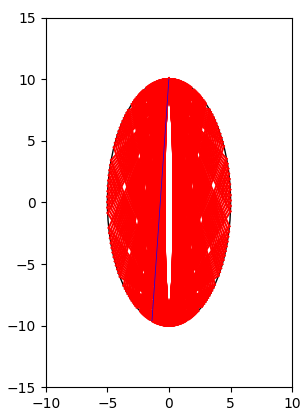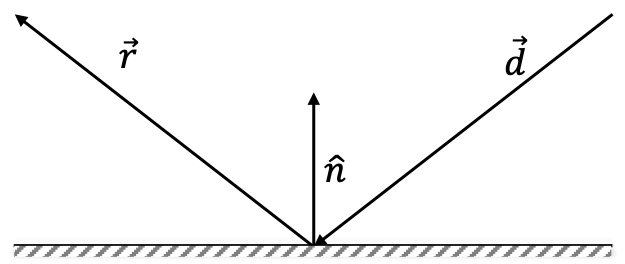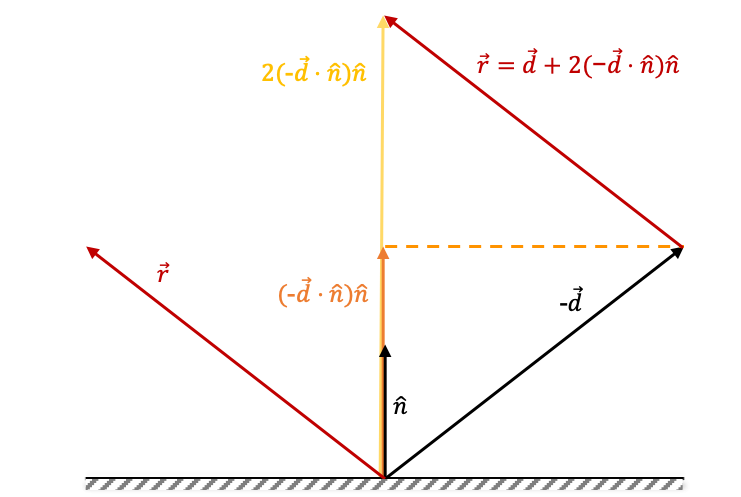# Reflections of a laser beam

25 Oct 2019

I must admit, I didn’t take the optics course during my Bs.c. in Physics. Nevertheless, One can never forget how light simply works - it hits the surface, and then reflects.

Project Euler problem 144 discuss the following problem (in short):

A lazer beam enters an ellipse ($4x^2 + y^2 = 100$) with small hole at it’s top ($−0.01\leq x\leq+0.01$) How many times does the beam hit the internal surface of the ellipse before exiting?For the specific details, you can read the full problem. Coming to solve this, vectors arithmetic comes in handy. I don’t know why this problem difficulty is 50%, given that any high-school graduate supposes to have the tools for it.

I wrote my solution in Python and used matplotlib for the animation. I won’t post it since it’s against ProjectEuler’s wish - solving the problem by yourself makes all the fun. This is the ellipse after %d steps. You can see the blue beam being the last to leave.I will, however, solve here my version of this problem, using a different shape.

A laser beam enters a rectangle with a small hole at its top (2px wide). How many times does the beam hit the internal surface of the ellipse before exiting?

when approaching this problem we need to answer a few questions:

1. How to detect where the laser beam hits?
2. How is the beam reflected after hitting the surface?

let’s answer it, step by step.

# How to detect where the laser beam hits?

We have a rectangle here, which has its pros and cons: on one side, it’s easier, since it’s composed out of 4 line segments. On the other, unlike the ellipse, it doesn’t have a single elegant equation, which would come in handy if we would like to detect the intersection between the beam and the shape. So, we have 4 line segments (rectangle) + 1 line segment (beam). * Let’s explore some lines segments intersection detection methods:

We would implement the method explained in the first link: given two lines, where each line has its starts and finish points, calculate using a parameter when the two would intersect.

The code is written in JavaScript, and we’ll use it later on in the simulation code:

var determinant = function(a, b, c, d) {
return a*d - b*c;
}

var doLinesIntesect = function(line1, line2) {
// calculations from https://en.wikipedia.org/wiki/Line–line_intersection
const x1 = line1;
const y1 = line1;
const x2 = line1;
const y2 = line1;
const x3 = line2;
const y3 = line2;
const x4 = line2;
const y4 = line2;

// When the two lines are parallel or coincident the denominator is zero:
const denominator = determinant(x1-x2, x3-x4, y1-y2, y3-y4);
if (denominator === 0) {
return [];
}

// The intersection point falls within the first line segment
// if 0.0 <= t <= 1.0
const t = determinant(x1-x3, x3-x4, y1-y3, y3-y4) / denominator;
if (t < 0 || 1 < t) {
return [];
}
const px = x1 + t * (x2-x1);
const py = y1 + t * (y2-y1);
return [px, py];
}


A beam would have 2 intersecting edges: the edge it came from and the edge it hits. A simple condition will give us the correct edge the beam hits.

# How is the beam reflected after hitting the surface?

Given an edge and a hitting beam, we would like to calculate the reflected beam. Converting each line to vector and solve it using the reflection formula is really simple and easy.Given $\hat{n}$, the normalized normal of the edge, pointed to the interior or the rectangle, and $\overrightarrow{d}$ the hitting beam vector, the reflected vector is described as:

That formula is really easy to grasp after writing down the vectors.JS code:

var getRefelctedVector = function(n, d) {
// d is the hitting vector
// n is the normalized normal
// r = d - 2(d*n)n, where * is dot product
rx = d - 2*(d*n+d*n)*n
ry = d - 2*(d*n+d*n)*n
return [rx, ry]
}

var getNextBeam = function(ctx, beam, startingEdge, edges, normals, limit) {
// calculate where the beam (vector d) hits the next edge.
var res =  getIntersection(startingEdge, beam, edges);
var n = normals[res.edgeIndex];
var d = getVectorDirection(beam);
var r = getRefelctedVector(n, d);

var newBeam = getLine(res.intesection, r, limit);
var normal = getLine(res.intesection, n, limit);

return [newBeam, res.edgeIndex];
}


The function gets the beam and starting edge, then calculates the vectors $\hat{n}$, $\overrightarrow{d}$, and then using linear algebra to calculate the reflected vector $\overrightarrow{r}$. Notice that $\hat{n}, \overrightarrow{d}, \overrightarrow{r}$ are all direction vectors (meaning they don’t reflect the real position on the grid). To get the segment line representation (point1->point2), we would combine the intersection point as the starting point, to the direction vector.

Since the Normal is not defined at corners, we would ignore that case. Also, since our system is discrete - we won’t observe this kind of behavior.

# Reflections inside a Rectangle

This is a simulation of a ray inside a rectangle. The ray enters from a hole in the middle of the top edge. You can find the source here.

Your browser does not support the HTML5 canvas tag.
#Steps 0
• Show beam
• Show Reflected Beam
• Show Normal
• Run Forever (ignore hole)
• Slow or Fast
• Starting Angle [0.1°-179.9°]45
• Max Steps (0 for inf):